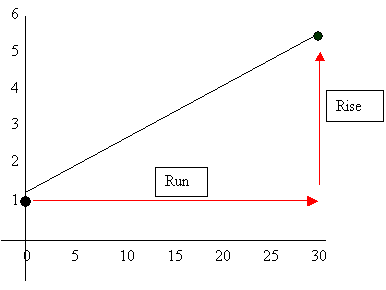Calculating slope intercept

The first thing you must do in order to calculate slope intercept is to calculate slope, (m). This can be done in a couple of ways. Slope is essentially the rise over run of a line in a graph. For instance if you take our example of age vs. height graph a best fit line was used.Calculate the coordinate points for the two farthest points on your line in this case (0, 1) for the first point and (30, 5.5) for the second point. Plug these points into the equation.

 m = y2 – y1   =  5.5 – 1  =  .15        x2 – x1        30 - 0

The next thing you want to do is plug it into the Y intercept equation

 Y = mx + b b is where the line intercepts the Y axis.  In this case it is at 1.

You can now take any X coordinate and place it into the equation and find your Y coordinate. For instance, place 15 for you’re X. You therefore get Y = (.15)15 + 1, solving for Y the answer us 3.25 which when you check on the graph is correct.